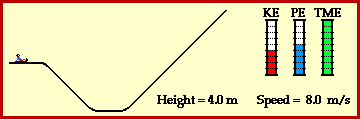## How High Will It Go?

The animation below depicts the motion of a young child sliding across the snow on a sled (combined mass of 50 kg). The child comes to a small decline to a valley below which is immediately followed by a steep incline. The child begins the descent down the decline with a speed of 8 m/s. If the snow is assumed to be friction free, then how high along the opposite incline will the child slide before eventually coming to a stop? And what variables will influence the answer? Would a change in the child's mass affect the height achieved on the opposite incline? Would a change in the angles of the decline and the incline affect the height achieved on the the opposite incline? Would a change in the child's speed affect the height achieved on the opposite incline?

The motion of the sledder in the animation below is similar to the motion of a roller coaster car on a roller coaster track. The similarity of the two motions is associated with the work-energy relationship. The energy bar charts accompanying the animation depict this relationship. Energy bar charts are a conceptual tool amount the amount of each form of energy possessed by an object as it an undergoes a particular motion. Observe from the animation that the total mechanical energy (TME) of the sledder remains constant throughout the motion. The total mechanical energy is the sum of the two forms of mechanical energy - kinetic energy (KE) and potential energy (PE). While the individual amounts of kinetic and potential energy are undergoing change, their sum will always be the same amount. As on a roller coaster, energy is transformed from potential energy to kinetic energy and vice versa. Provided that external forces (such as friction forces and applied forces) do not do work, the total amount of mechanical energy will be held constant.In the initial state on top of the hill, the sledder has both kinetic (energy of motion) and potential energy (energy of vertical position). The amount of kinetic energy is dependent upon mass and speed, and computed from the equation KE=0.5*m*v2. For the 50-kg sledder (includes the sled's mass) moving at 8.0 m/s, the kinetic energy is 1600 Joules. The amount of potential energy is dependent upon mass and height and is found using the equation PE=m*g*h where g is the acceleration of gravity (approximated here to be 10 m/s/s). For a 50-kg sledder on top of a 4.0-meter high hill, the potential energy is 2000 Joules. The total amount of mechanical energy (kinetic plus potential) is then 3600 J. Since this quantity of mechanical energy will be conserved, the sledder must still have 3600 Joules of mechanical energy by the time he/she comes to a stop on the opposite incline. At that instant, all 3600 Joules of mechanical energy will be in the form of potential energy. The height of the sledder can then be calculated using the equation PE = m*g*h where PE = 3600 J, m = 50 kg, and g = 10 m/s/s (the same approximation as used earlier). The substitution and algebra yields an answer of 7.2 meters.

Note that the solution to the above problem does not take into account the angles of the decline and incline. The angles would only have an affect upon the acceleration of the sledder along the hills. A steeper incline would be consistent with a greater acceleration value; yet this greater acceleration would occur for less time, thus yielding the same speed at the bottom of the hill and the same final height on the opposite incline. Thus the angles of the decline and incline have no affect upon the final height achieved by the sledder.

But perhaps the mass of the sledder would have an affect upon the final height which the sledder would achieve? After all, the mass of the sledder does enter into the equations. To investigate the affect of mass upon the scenario, perform the same calculations as above for an 80-kg sledder with the same initial height of 4.0 meters and the same initial speed of 8.0 m/s. Use the pull down menu to check each individual answer.

1. Determine the initial kinetic energy of the 80-kg sledder.

2. Determine the initial potential energy of the 80-kg sledder.

3. Determine the initial mechanical energy of the 80-kg sledder.

4. Determine the mechanical energy possessed by the sledder when he/she comes to a stop at the final height.

5. When finally stopped on the opposite incline, how much potential energy will the sledder have?

6. Determine the final height achieved by this more massive (80 kg) sledder?

7. Does the mass of the sledder have an affect upon the height achieved by the sledder?

For more information on physical descriptions of motion, visit The Physics Classroom Tutorial. Detailed information is available there on the following topics:

Potential Energy

Kinetic Energy

Mechanical Energy

Internal vs. External Forces

Work

Work-Energy Theorem

Work-Energy Bar Charts